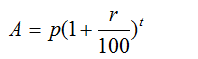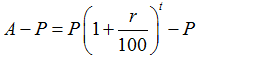### Compound Interest Definition, Formula, Concepts, Examples and Practice Questions: The Complete Lessons

Welcome to this lesson on Compound Interest

Compound Interest is an extremely important part of Arithmetic/Commercial mathematics. In this lesson, we cover all the concepts for Compound Interest and provide you all the possible information you require for the topic.

Over the course of 4 articles, we cover each and every aspect related to Compound Interest. We cover the following the articles:

• What is Compound Interest?
• Applications of Compound Interest including different problem types.
• Negative Compound Interest
• Useful Shortcuts and tricks for Compound Interest

### Compound Interest Definition

The compound interest definition is as follows: Compound interest (also known compounding interest) is the interest amount calculated on the initial principal (this is the original amount of a loan on which interest is calculated) and also on the accrued interest of previous periods of a deposit/loan. Compounding of interest is a common practice in most financial transactions. In fact, all bank transactions are based on compound interest.

Compound Interest Formula
The first step is to find the amount due at the end of compounding time period.
In the formula:
A: Final Amount
P: Principal (original amount of sum)
R: Rate of Interest (in %)
T: Time period (yearly, half-yearly etc.)

The formula for the final amount is:To find the Compound Interest over the time period T, we need to subtract the original amount from the final amount. The compound interest is give by by the formula:This can also be written as:
Compound Interest= P {(1+r/100)t -1}

In order to learn further details for the topic, you should head over the first article for the topic and start your learning. Use the table of contents below to start your learning journey with us.